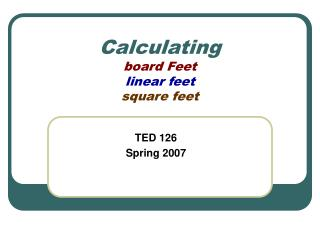DownloadDownload PresentationCalculating board Feet linear feet square feet

# Calculating board Feet linear feet square feet

Download Presentation## Calculating board Feet linear feet square feet

- - - - - - - - - - - - - - - - - - - - - - - - - - - E N D - - - - - - - - - - - - - - - - - - - - - - - - - - -
##### Presentation Transcript

1. Calculatingboard Feetlinear feetsquare feet TED 126 Spring 2007

2. Board Feet • "Board Feet" is a measurement of lumber volume. • A board foot is equal to 144 cubic inches of wood. • Actually it's easy to calculate using the following formula: Bd. Ft. =T (inches) x W (inches) x L (feet) / 12

3. Board Feet • When you are figuring up board feet, keep in mind a waste factor. • If you purchase good clear material add about 15% for waste, • if you elect to use lower grade material you will have to allow for defects and more wasted material ---add about 30%.

4. Board Feet Bd. Ft. = T (inches) x W (inches) x L (feet) / 12 Bd. Ft. = T (inches) x W (inches) x L (inches) / 144

5. Board Feet and Linear feet • A linear foot is a measure of length 12 inches long and a • board foot is a number calculated by determining the volume of a board that is 12 inches wide and 1 inch thick. • In other words, a 1" x 6" board that measures 24" long is exactly one board foot. (width" x thickness" x length' / 12)

6. Board Feet and Linear feet • To convert linear feet to board feet:Thickness” x Width” x Length’ ÷ 12 • To convert board feet to linear feet: 12 ÷ Thickness” x Width” x Board Foot

7. Linear feet andSquare Feet • A Linear Feet is just a measurement of length and does not take into account its width or thickness. • Square Feetmeasures area, simply width x length.

8. The math…. • It is not possible to convert linear footageintosquare footage because a linear foot is only one dimension and a square foot is two dimensions, • and additionally, a "board foot" is an example of three dimensions. • However, if you know the linear footage as well as the width, you can divide the width by 12 and multiply by the linear footage - that should give you the square footage of a particular bundle of flooring. • To convertsquare feettolinear feet:square feet / width of board in feet = linear feet

9. Square Foot • SQUARE FOOTAGE – A unit of measurement to determine that total amount of flooring required for a given area. It is how much surface a milled wood product will cover. • In practice, calculate by multiplying the length times the width of the space. • For the purpose of determining the amount of square footage per width in a given flooring bundle, • calculate by dividing the width of the flooring by 12 and then multiplying be the total linear footage (length of layers x number of layers)."

10. Materials… • Plywood, hardboard, particle board and other sheet material are sold by the Square foot. • Moldings, trim, dowel rods, wood siding / paneling and similar materials are sold by the Linear foot.

11. Siding example… • Redwood Siding and paneling is sold by the linear (or lineal) foot. Your quantity requirements are calculated by multiplying the square foot area by a factor based on the siding width. • A) Calculate the square footage in walls: length x height = sq. ft. B) Calculate, and then subtract, the square footage of openings C) Add 10% to cover trim and waste D) Multiply the result by the factor for lineal feet • For example: If you are paneling four walls 8 feet high by 40 feet long with 1x6" Tongue and Groove redwood siding and we allow 10% of the total square feet for openings, you would need approximately 3300-3400 linear feet: • 4 * 8 * 40 = 1280 SQ FT - 128 (10% openings) + 115 (10% trim/waste) = 1267 SQ FT * 2.67 linear factor = 3383 LFT THE END